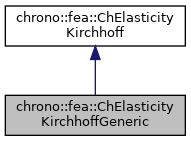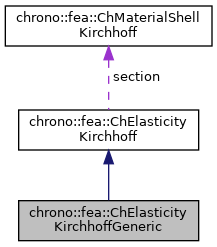chrono::fea::ChElasticityKirchhoffGeneric Class Reference

## Description

Generic linear elasticity for thin shells (Kirchoff-Love shell theory, without shear effects) to be used in a ChMaterialShellKirchhoff.

This uses a 6x6 matrix [E] from user-input data. The [E] matrix can be computed from a preprocessing stage using a FEA analysis over a detailed 3D model of a slab of shell, hence recovering the 6x6 matrix in the linear mapping: {n,m}=[E]{e,k}.

#include <ChMaterialShellKirchhoff.h>

Inheritance diagram for chrono::fea::ChElasticityKirchhoffGeneric:[legend]
Collaboration diagram for chrono::fea::ChElasticityKirchhoffGeneric:[legend]

## Public Member Functions

ChMatrixNM< double, 6, 6 > & Ematrix ()
Access the E matrix, for getting/setting its values. More...

virtual void ComputeStress (ChVector<> &n, ChVector<> &m, const ChVector<> &eps, const ChVector<> &kur, const double z_inf, const double z_sup, const double angle) override
The FE code will evaluate this function to compute per-unit-length forces/torques given the strains/curvatures. More...

virtual void ComputeStiffnessMatrix (ChMatrixRef mC, const ChVector<> &eps, const ChVector<> &kur, const double z_inf, const double z_sup, const double angle) override
Compute 6x6 stiffness matrix [Km] , that is [ds/de], the tangent of the constitutive relation per-unit-length forces/torques vs generalized strains. More...Public Attributes inherited from chrono::fea::ChElasticityKirchhoff
ChMaterialShellKirchhoffsection

## ◆ ComputeStiffnessMatrix()

 void chrono::fea::ChElasticityKirchhoffGeneric::ComputeStiffnessMatrix ( ChMatrixRef mC, const ChVector<> & eps, const ChVector<> & kur, const double z_inf, const double z_sup, const double angle )
overridevirtual

Compute 6x6 stiffness matrix [Km] , that is [ds/de], the tangent of the constitutive relation per-unit-length forces/torques vs generalized strains.

Parameters
 mC tangent matrix eps strains e_11, e_22, e_12 kur curvature k_11, k_22, k_12 z_inf layer lower z value (along thickness coord) z_sup layer upper z value (along thickness coord) angle layer angle respect to x (if needed)

Reimplemented from chrono::fea::ChElasticityKirchhoff.

## ◆ ComputeStress()

 void chrono::fea::ChElasticityKirchhoffGeneric::ComputeStress ( ChVector<> & n, ChVector<> & m, const ChVector<> & eps, const ChVector<> & kur, const double z_inf, const double z_sup, const double angle )
overridevirtual

The FE code will evaluate this function to compute per-unit-length forces/torques given the strains/curvatures.

Parameters
 n forces n_11, n_22, n_12 (per unit length) m torques m_11, m_22, m_12 (per unit length) eps strains e_11, e_22, e_12 kur curvature k_11, k_22, k_12 z_inf layer lower z value (along thickness coord) z_sup layer upper z value (along thickness coord) angle layer angle respect to x (if needed) -not used in this, isotropic

Implements chrono::fea::ChElasticityKirchhoff.

## ◆ Ematrix()

 ChMatrixNM& chrono::fea::ChElasticityKirchhoffGeneric::Ematrix ( )
inline

Access the E matrix, for getting/setting its values.

This is the matrix that defines the linear elastic constitutive model as it maps yxz displacements "e" and xyz rotations "k" to the "n" force and "m" torque as in {n,m}=[E]{e,k}.

The documentation for this class was generated from the following files:
• /builds/uwsbel/chrono/src/chrono/fea/ChMaterialShellKirchhoff.h
• /builds/uwsbel/chrono/src/chrono/fea/ChMaterialShellKirchhoff.cpp